FreeFEM Documentation on GitHub

stars - forks

# Tutorial

Authors: Pierre-Henri Tournier - Frédéric Nataf - Pierre Jolivet

We recommend checking the slides version of this tutorial.

## What is ffddm ?

• ffddm implements a class of parallel solvers in FreeFEM: overlapping Schwarz domain decomposition methods

• The entire ffddm framework is written in the FreeFEM language ffddm aims at simplifying the use of parallel solvers in FreeFEM

You can find the ffddm scripts here (‘ffddm*.idp’ files) and examples here

• ffddm provides a set of high-level macros and functions to
• handle data distribution: distributed meshes and linear algebra

• build DD preconditioners for your variational problems

• solve your problem using preconditioned Krylov methods

• ffddm implements scalable two level Schwarz methods, with a coarse space correction built either from a coarse mesh or a GenEO coarse space Ongoing research: approximate coarse solves and three level methods

• ffddm can also act as a wrapper for the HPDDM library.

HPDDM is an efficient C++11 implementation of various domain decomposition methods and Krylov subspace algorithms with advanced block and recycling techniques More details on how to use HPDDM within ffddm here

## Why Domain Decomposition Methods ?

How can we solve a large sparse linear system $$A u = b \in \mathbb{R}^n$$ ?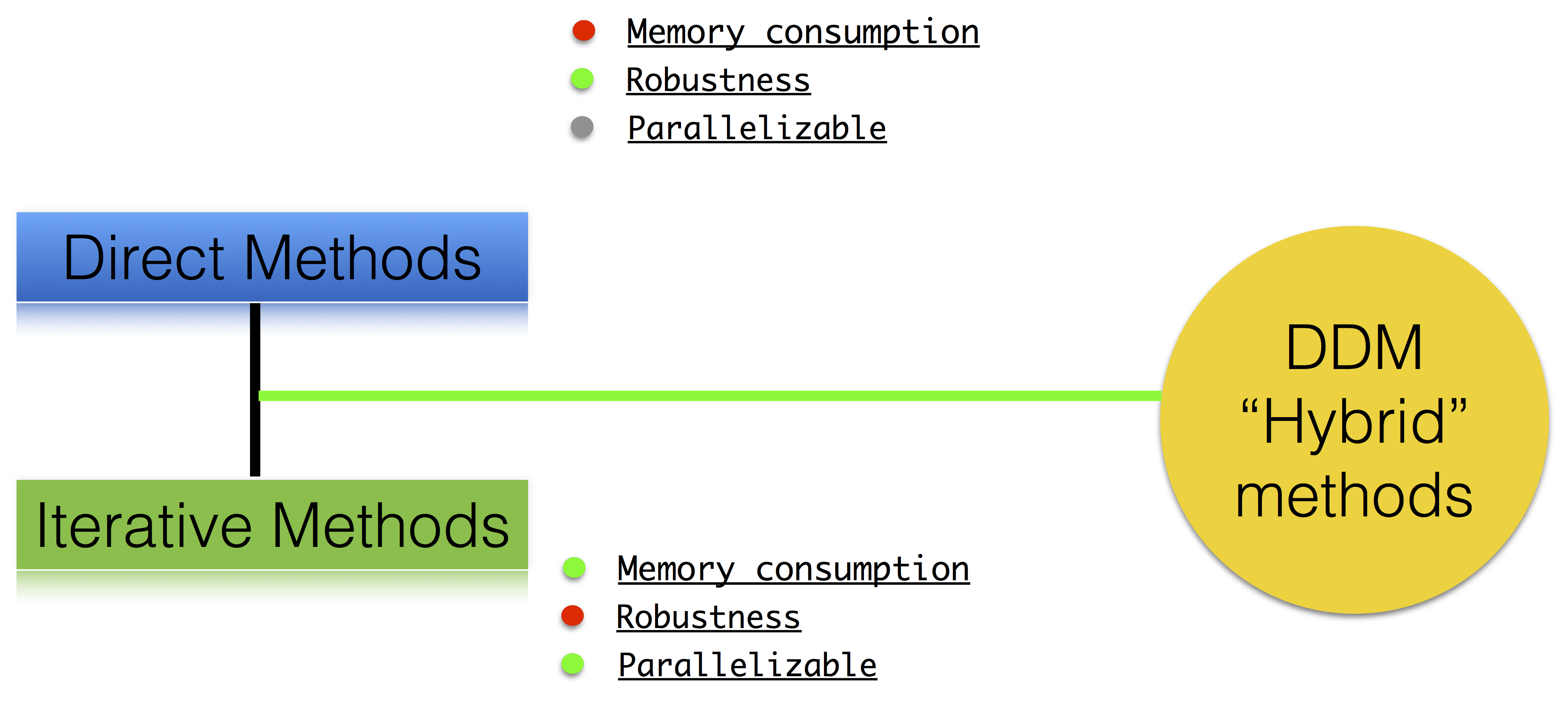### Step 1: Decompose the mesh

Build a collection of $$N$$ overlapping sub-meshes $$(Th_{i})_{i=1}^N$$ from the global mesh $$Th$$

1ffddmbuildDmesh( prmesh , ThGlobal , comm )

• mesh distributed over the MPI processes of communicator comm

• initial mesh ThGlobal partitioned with metis by default

• size of the overlap given by ffddmoverlap (default 1)

prmesh#Thi is the local mesh of the subdomain for each mpi process

 1macro dimension 2// EOM            // 2D or 3D
2
3include "ffddm.idp"
4
5mesh ThGlobal = square(100,100);    // global mesh
6
7// Step 1: Decompose the mesh
8ffddmbuildDmesh( M , ThGlobal , mpiCommWorld )
9
10medit("Th"+mpirank, MThi);


Copy and paste this to a file ‘test.edp’ and run it:

1ff-mpirun -np 2 test.edp -wg


### Step 2: Define your finite element

1ffddmbuildDfespace( prfe , prmesh , scalar , def , init , Pk )


builds the local finite element spaces and associated distributed operators on top of the mesh decomposition prmesh

• scalar: type of data for this finite element: real or complex

• Pk: your type of finite element: P1, [P2,P2,P1], …

• def, init: macros specifying how to define and initialize a Pk FE function

prfe#Vhi is the local FE space defined on prmesh#Thi for each mpi process

Example for P2 complex:

1macro def(u)  u // EOM
2macro init(u) u // EOM
3ffddmbuildDfespace( FE, M, complex,
4                    def, init, P2 )


Example for [P2,P2,P1] real:

1macro def(u)  [u, u#B, u#C] // EOM
2macro init(u) [u, u, u]     // EOM
3ffddmbuildDfespace( FE, M, real, def,
4                    init, [P2,P2,P1] )


#### Distributed vectors and restriction operators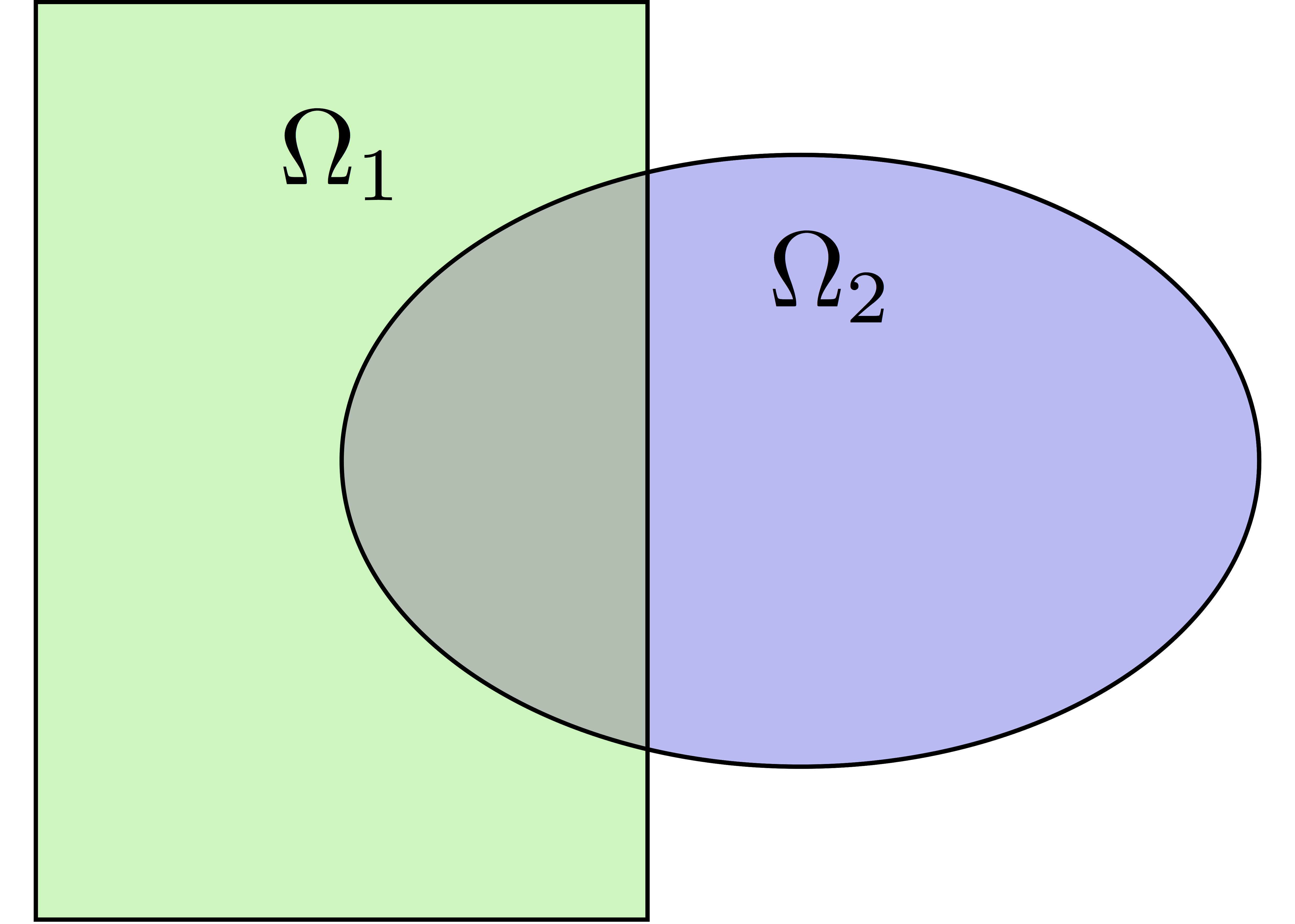Natural decomposition of the set of d.o.f.’s $${\mathcal N}$$ of $$Vh$$ into the $$N$$ subsets of d.o.f.’s $$({\mathcal N}_i)_{i=1}^N$$ each associated with the local FE space $$Vh_i$$

${\mathcal N} = \cup_{i=1}^N {\mathcal N}_i\,,$

but with duplications of the d.o.f.’s in the overlap

_Definition_ a distributed vector is a collection of local vectors $$({\mathbf V_i})_{1\le i\le N}$$ so that the values on the duplicated d.o.f.’s are the same:

${\mathbf V}_i = R_i\,{\mathbf V}, \quad i = 1, ..., N$

where $${\mathbf V}$$ is the corresponding global vector and $$R_i$$ is the restriction operator from $${\mathcal N}$$ into $${\mathcal N}_i$$

Remark $$R_i^T$$ is the extension operator: extension by $$0$$ from $${\mathcal N}_i$$ into $${\mathcal N}$$

#### Partition of unity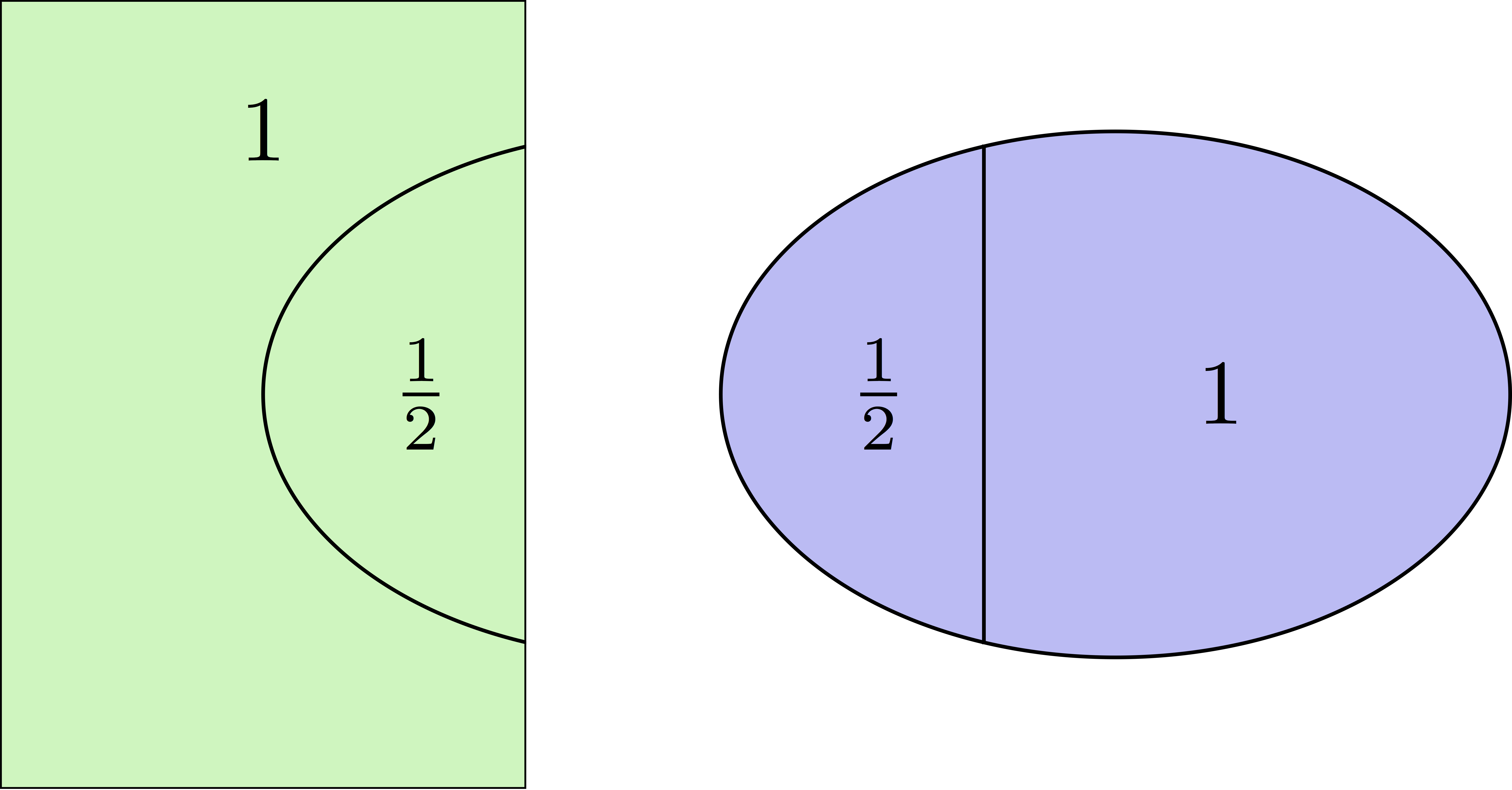Duplicated unknowns coupled via a partition of unity:

$I = \sum_{i = 1}^N R_i^T D_i R_i$

$$(D_i)_{1\le i \le N}$$ are square diagonal matrices of size $$\#{\mathcal N}_i$$

${\mathbf V} = \sum_{i = 1}^N R_i^T D_i R_i {\mathbf V} = \sum_{i = 1}^N R_i^T D_i {\mathbf V_i}$

#### Data exchange between neighbors

1func prfe#update(K[int] vi, bool scale)


synchronizes local vectors $${\mathbf V}_i$$ between subdomains $$\Rightarrow$$ exchange the values of $$mathbf{V}_i$$ shared with neighbors in the overlap region

${\mathbf V}_i \leftarrow R_i \left( \sum_{j=1}^N R_j^T D_j {\mathbf V}_j \right) = D_i {\mathbf V}_i + \sum_{j\in \mathcal{O}(i)} R_i\,R_j^T\,D_j {\mathbf V}_j$

where $$\mathcal{O}(i)$$ is the set of neighbors of subdomain $i$. Exchange operators $$R_i\,R_j^T$$ correspond to neighbor-to-neighbor MPI communications

1FEupdate(vi, false);

${\mathbf V}_i \leftarrow R_i \left( \sum_{j=1}^N R_j^T {\mathbf V}_j \right)$
1FEupdate(vi, true);

${\mathbf V}_i \leftarrow R_i \left( \sum_{j=1}^N R_j^T D_j {\mathbf V}_j \right)$
 1macro dimension 2// EOM            // 2D or 3D
2
3include "ffddm.idp"
4
5mesh ThGlobal = square(100,100);    // global mesh
6
7// Step 1: Decompose the mesh
8ffddmbuildDmesh( M , ThGlobal , mpiCommWorld )
9
10// Step 2: Define your finite element
11macro def(u)  u // EOM
12macro init(u) u // EOM
13ffddmbuildDfespace( FE , M , real , def , init , P2 )
14
15FEVhi vi = x;
16medit("v"+mpirank, MThi, vi);
17
18vi[] = FEDk[mpirank];
19medit("D"+mpirank, MThi, vi);
20
21vi = 1;
22FEupdate(vi[],true);
23ffddmplot(FE,vi,"1")
24
25FEupdate(vi[],false);
26ffddmplot(FE,vi,"multiplicity")


### Step 3: Define your problem

1ffddmsetupOperator( pr , prfe , Varf )


builds the distributed operator associated to your variational form on top of the distributed FE prfe

Varf is a macro defining your abstract variational form

1macro Varf(varfName, meshName, VhName)
3                       + int2d(meshName)(f*v) + on(1, u = 0);  // EOM


$$\Rightarrow$$ assemble local ‘Dirichlet’ matrices $$A_i = R_i A R_i^T$$

$A = \sum_{i=1}^N R_i^T D_i A_i R_i$

Warning

only true because $$D_i R_i A = D_i A_i R_i$$ due to the fact that $$D_i$$ vanishes at the interface !!

pr#A applies $$A$$ to a distributed vector: $${\mathbf U}_i \leftarrow R_i \sum_{j=1}^N R_j^T D_j A_j {\mathbf V}_j$$

$$\Rightarrow$$ multiply by $$A_i$$ + prfe#update

 1macro dimension 2// EOM            // 2D or 3D
2
3include "ffddm.idp"
4
5mesh ThGlobal = square(100,100);    // global mesh
6
7// Step 1: Decompose the mesh
8ffddmbuildDmesh( M , ThGlobal , mpiCommWorld )
9
10// Step 2: Define your finite element
11macro def(u)  u // EOM
12macro init(u) u // EOM
13ffddmbuildDfespace( FE , M , real , def , init , P2 )
14
15// Step 3: Define your problem
16macro grad(u) [dx(u), dy(u)] // EOM
17macro Varf(varfName, meshName, VhName)
19                       + int2d(meshName)(1*v) + on(1, u = 0);  // EOM
20ffddmsetupOperator( PB , FE , Varf )
21
22FEVhi ui, bi;
23ffddmbuildrhs( PB , Varf , bi[] )
24
25ui[] = PBA(bi[]);
26ffddmplot(FE, ui, "A*b")


## Summary so far: translating your sequential FreeFEM script

### Step 1: Decompose the mesh

1mesh Th = square(100,100);

1mesh Th = square(100,100);
2ffddmbuildDmesh(M, Th, mpiCommWorld)


Step 2: Define your finite element

1fespace Vh(Th, P1);

1macro def(u)  u // EOM
2macro init(u) u // EOM
3ffddmbuildDfespace(FE, M, real, def, init, P1)


1varf Pb(u, v) = ...
2matrix A = Pb(Vh, Vh);

1macro Varf(varfName, meshName, VhName)
2    varf varfName(u,v) = ... // EOM
3ffddmsetupOperator(PB, FE, Varf)


### Solve the linear system

1u[] = A^-1 * b[];

1ui[] = PBdirectsolve(bi[]);


### Solve the linear system with the parallel direct solver MUMPS

1func K[int] pr#directsolve(K[int]& bi)


We have $$A$$ and $$b$$ in distributed form, we can solve the linear system $$A u = b$$ using the parallel direct solver MUMPS

1// Solve the problem using the direct parallel solver MUMPS
2ui[] = PBdirectsolve(bi[]);
3ffddmplot(FE, ui, "u")


### Step 4: Define the one level DD preconditioner

1ffddmsetupPrecond( pr , VarfPrec )


builds the one level preconditioner for problem pr.

By default it is the Restricted Additive Schwarz (RAS) preconditioner:

$M^{-1}_1 = M^{-1}_{\text{RAS}} = \sum_{i=1}^N R_i^T D_i A_i^{-1} R_i \quad \text{with}\; A_i = R_i A R_i^T$

_Setup step_: compute the $$LU$$ (or $$L D L^T$$) factorization of local matrices $$A_i$$

pr#PREC1 applies $$M^{-1}_1$$ to a distributed vector: $${\mathbf U}_i \leftarrow R_i \sum_{j=1}^N R_j^T D_j A_j^{-1} {\mathbf V}_i$$

$$\Rightarrow$$ apply $$A_i^{-1}$$ (forward/backward substitutions) + prfe#update

### Step 5: Solve the linear system with preconditioned GMRES

1func K[int] pr#fGMRES(K[int]& x0i, K[int]& bi, real eps, int itmax, string sp)


solves the linear system with flexible GMRES with DD preconditioner $$M^{-1}$$

• x0i: initial guess

• bi: right-hand side

• eps: relative tolerance

• itmax: maximum number of iterations

• sp: “left” or “right” preconditioning

left preconditioning

solve $$M^{-1} A x = M^{-1} b$$

right preconditioning

solve $$A M^{-1} y = b$$

$$\Rightarrow x = M^{-1} y$$

 1macro dimension 2// EOM            // 2D or 3D
2include "ffddm.idp"
3
4mesh ThGlobal = square(100,100);    // global mesh
5// Step 1: Decompose the mesh
6ffddmbuildDmesh( M , ThGlobal , mpiCommWorld )
7// Step 2: Define your finite element
8macro def(u)  u // EOM
9macro init(u) u // EOM
10ffddmbuildDfespace( FE , M , real , def , init , P2 )
11// Step 3: Define your problem
12macro grad(u) [dx(u), dy(u)] // EOM
13macro Varf(varfName, meshName, VhName)
15                       + int2d(meshName)(1*v) + on(1, u = 0);  // EOM
16ffddmsetupOperator( PB , FE , Varf )
17
18FEVhi ui, bi;
19ffddmbuildrhs( PB , Varf , bi[] )
20
21// Step 4: Define the one level DD preconditioner
22ffddmsetupPrecond( PB , Varf )
23
24// Step 5: Solve the linear system with GMRES
25FEVhi x0i = 0;
26ui[] = PBfGMRES(x0i[], bi[], 1.e-6, 200, "right");
27
28ffddmplot(FE, ui, "u")
29PBwritesummary


## Define a two level DD preconditioner

Goal improve scalability of the one level method

$$\Rightarrow$$ enrich the one level preconditioner with a coarse problem coupling all subdomains

Main ingredient is a rectangular matrix $$\color{red}{Z}$$ of size $$n \times n_c,\,$$ where $$n_c \ll n$$ $$\color{red}{Z}$$ is the coarse space matrix

The coarse space operator $$E = \color{red}{Z}^T A \color{red}{Z}$$ is a square matrix of size $$n_c \times n_c$$

The simplest way to enrich the one level preconditioner is through the additive coarse correction formula:

$M^{-1}_2 = M^{-1}_1 + \color{red}{Z} E^{-1} \color{red}{Z}^T$

How to choose $$\color{red}{Z}$$ ?

## Build the GenEO coarse space

1ffddmgeneosetup( pr , Varf )


The GenEO method builds a robust coarse space for highly heterogeneous or anisotropic SPD problems

$$\Rightarrow$$ solve a local generalized eigenvalue problem in each subdomain

$D_i A_i D_i\, V_{i,k} = \lambda_{i,k}\, A_i^{\text{Neu}} \,V_{i,k}$

with $$A_i^{\text{Neu}}$$ the local Neumann matrices built from Varf (same Varf as Step 3)

The GenEO coarse space is $$\color{red}{Z} = (R_i^T D_i V_{i,k})^{i=1,...,N}_{\lambda_{i,k} \ge \color{blue}{\tau}}$$ The eigenvectors $$V_{i,k}$$ selected to enter the coarse space correspond to eigenvalues $$\lambda_{i,k} \ge \color{blue}{\tau}$$, where $$\color{blue}{\tau}$$ is a threshold parameter

Theorem the spectrum of the preconditioned operator lies in the interval $$[\displaystyle \frac{1}{1+k_1 \color{blue}{\tau}} , k_0 ]$$ where $$k_0 - 1$$ is the # of neighbors and $$k_1$$ is the multiplicity of intersections $$\Rightarrow$$ $$k_0$$ and $$k_1$$ do not depend on $$N$$ nor on the PDE

 1macro dimension 2// EOM            // 2D or 3D
2include "ffddm.idp"
3
4mesh ThGlobal = square(100,100);    // global mesh
5// Step 1: Decompose the mesh
6ffddmbuildDmesh( M , ThGlobal , mpiCommWorld )
7// Step 2: Define your finite element
8macro def(u)  u // EOM
9macro init(u) u // EOM
10ffddmbuildDfespace( FE , M , real , def , init , P2 )
11// Step 3: Define your problem
12macro grad(u) [dx(u), dy(u)] // EOM
13macro Varf(varfName, meshName, VhName)
15                       + int2d(meshName)(1*v) + on(1, u = 0);  // EOM
16ffddmsetupOperator( PB , FE , Varf )
17
18FEVhi ui, bi;
19ffddmbuildrhs( PB , Varf , bi[] )
20
21// Step 4: Define the one level DD preconditioner
22ffddmsetupPrecond( PB , Varf )
23
24// Build the GenEO coarse space
25ffddmgeneosetup( PB , Varf )
26
27// Step 5: Solve the linear system with GMRES
28FEVhi x0i = 0;
29ui[] = PBfGMRES(x0i[], bi[], 1.e-6, 200, "right");


## Build the coarse space from a coarse mesh

1ffddmcoarsemeshsetup( pr , Thc , VarfEprec , VarfAprec )


For non SPD problems, an alternative is to build the coarse space by discretizing the PDE on a coarser mesh Thc

$$Z$$ will be the interpolation matrix from the coarse FE space $${Vh}_c$$ to the original FE space $$Vh$$

$$\Rightarrow E=\color{red}{Z}^{T} A \color{red}{Z}$$ is the matrix of the problem discretized on the coarse mesh

The variational problem to be discretized on Thc is given by macro VarfEprec

VarfEprec can differ from the original Varf of the problem

Example: added absorption for wave propagation problems

Similarly, VarfAprec specifies the global operator involved in multiplicative coarse correction formulas. It defaults to $$A$$ if VarfAprec is not defined

 1macro dimension 2// EOM            // 2D or 3D
2include "ffddm.idp"
3
4mesh ThGlobal = square(100,100);    // global mesh
5// Step 1: Decompose the mesh
6ffddmbuildDmesh( M , ThGlobal , mpiCommWorld )
7// Step 2: Define your finite element
8macro def(u)  u // EOM
9macro init(u) u // EOM
10ffddmbuildDfespace( FE , M , real , def , init , P2 )
11// Step 3: Define your problem
12macro grad(u) [dx(u), dy(u)] // EOM
13macro Varf(varfName, meshName, VhName)
15                       + int2d(meshName)(1*v) + on(1, u = 0);  // EOM
16ffddmsetupOperator( PB , FE , Varf )
17
18FEVhi ui, bi;
19ffddmbuildrhs( PB , Varf , bi[] )
20
21// Step 4: Define the one level DD preconditioner
22ffddmsetupPrecond( PB , Varf )
23
24// Build the coarse space from a coarse mesh
25mesh Thc = square(10,10);
26ffddmcoarsemeshsetup( PB , Thc , Varf , null )
27
28// Step 5: Solve the linear system with GMRES
29FEVhi x0i = 0;
30ui[] = PBfGMRES(x0i[], bi[], 1.e-6, 200, "right");


## Use HPDDM within ffddm

ffddm allows you to use HPDDM to solve your problem, effectively replacing the ffddm implementation of all parallel linear algebra computations

$$\Rightarrow$$ define your problem with ffddm, solve it with HPDDM

$$\Rightarrow$$ ffddm acts as a finite element interface for HPDDM

You can use HPDDM features unavailable in ffddm such as advanced Krylov subspace methods implementing block and recycling techniques

To switch to HPDDM, simply define the macro pr#withhpddm before using ffddmsetupOperator (Step 3). You can then pass HPDDM options with command-line arguments or directly to the underlying HPDDM operator. Options need to be prefixed by the operator prefix:

1macro PBwithhpddm()1 // EOM
2ffddmsetupOperator( PB , FE , Varf )
3set(PBhpddmOP,sparams="-hpddm_PB_krylov_method gcrodr -hpddm_PB_recycle 10");


Or, define pr#withhpddmkrylov to use HPDDM only for the Krylov method

Example here: Helmholtz problem with multiple rhs solved with Block GMRES

## Some results: Heterogeneous 3D elasticity with GenEO

Heterogeneous 3D linear elasticity equation discretized with P2 FE solved on 4096 MPI processes $$n\approx$$ 262 million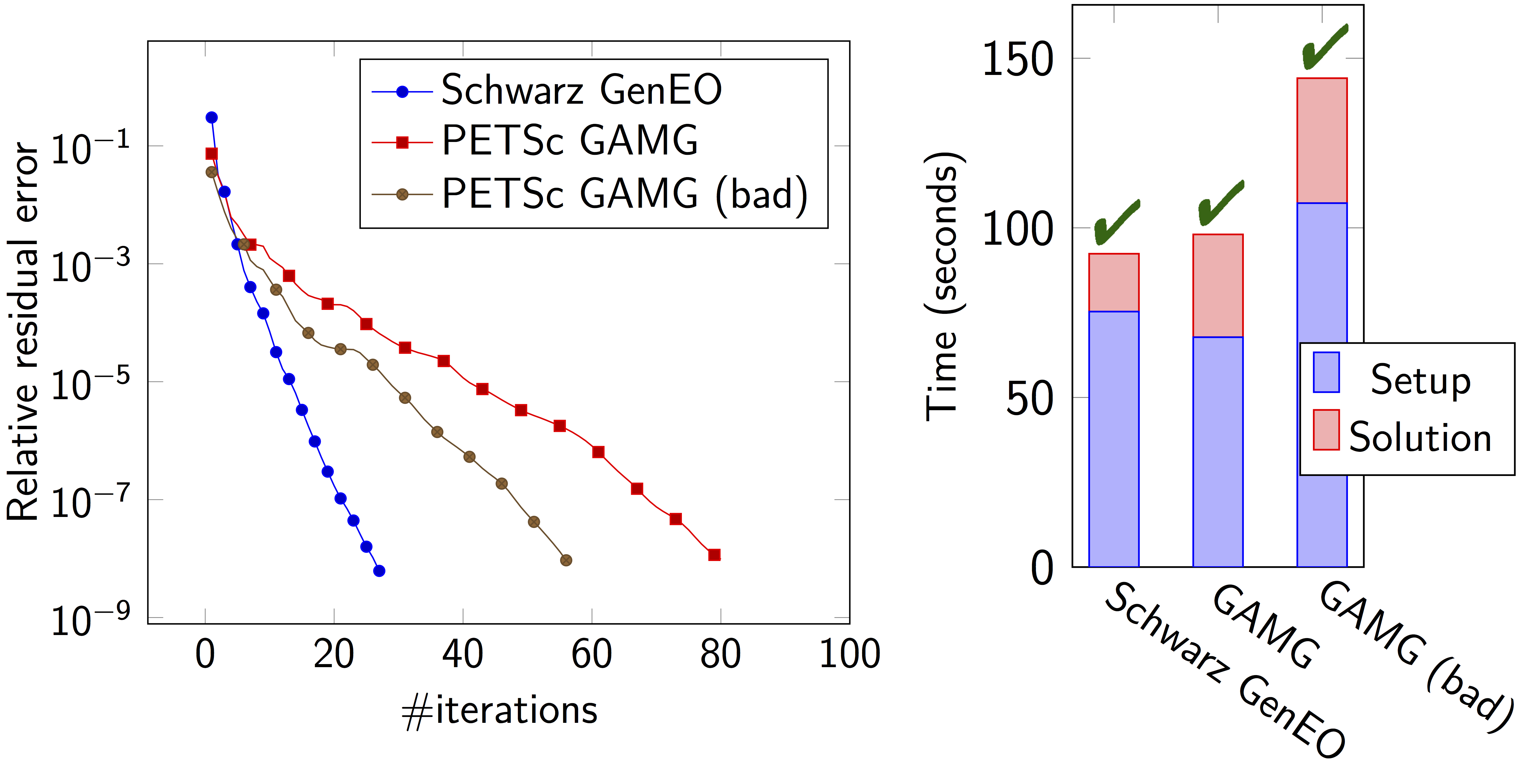## Some results: 2-level DD for Maxwell equations, scattering from the COBRA cavity

f = 10 GHz

f = 16 GHz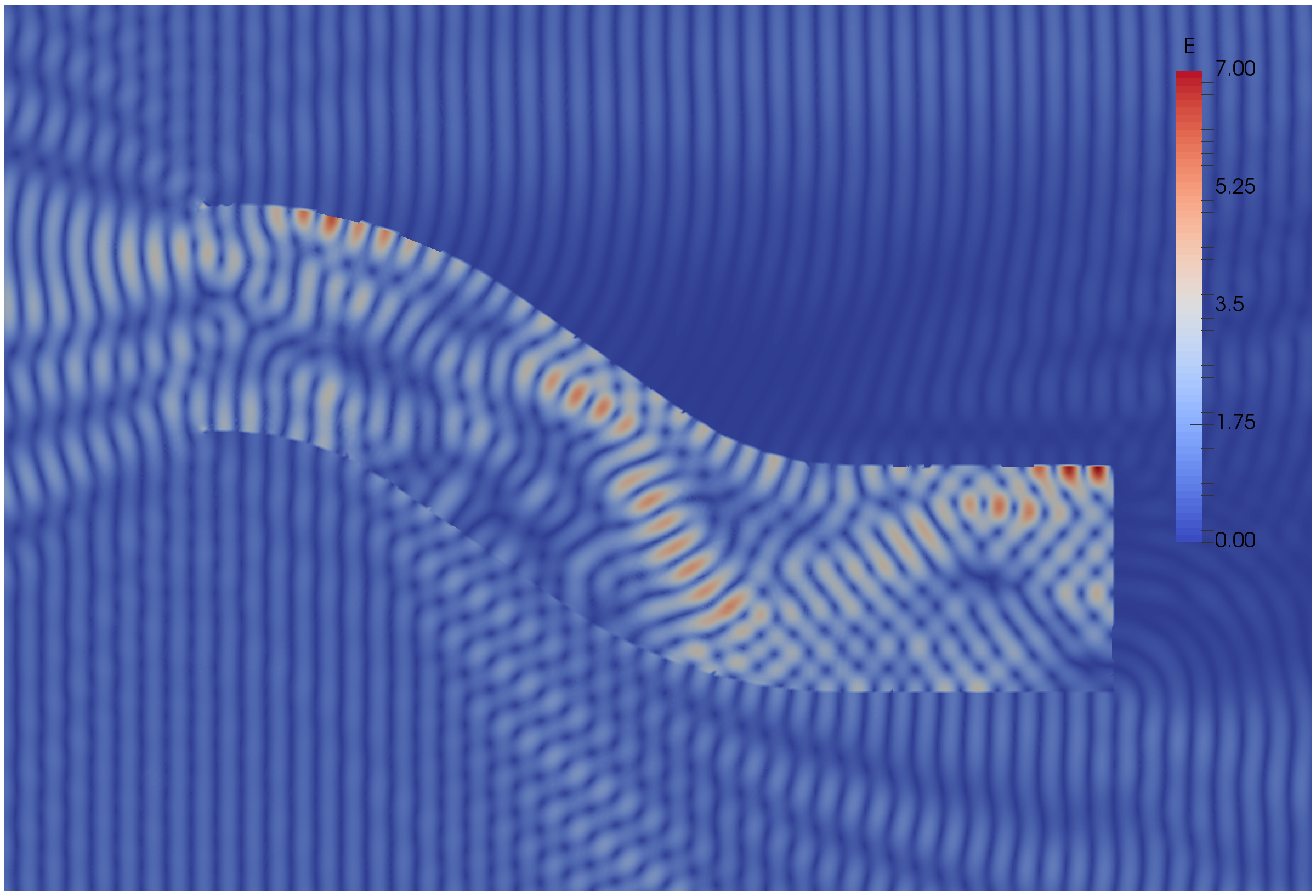## Some results: 2-level DD for Maxwell equations, scattering from the COBRA cavity

• order 2 Nedelec edge FE

• fine mesh: 10 points per wavelength

• coarse mesh: 3.33 points per wavelength

• two level ORAS preconditioner with added absorption

• f = 10 GHz: $$n\approx$$ 107 million, $$n_c \approx$$ 4 million

f = 16 GHz: $$n\approx$$ 198 million, $$n_c \approx$$ 7.4 million

$$\rightarrow$$ coarse problem too large for a direct solver $$\Rightarrow$$ inexact coarse solve: GMRES + one level ORAS preconditioner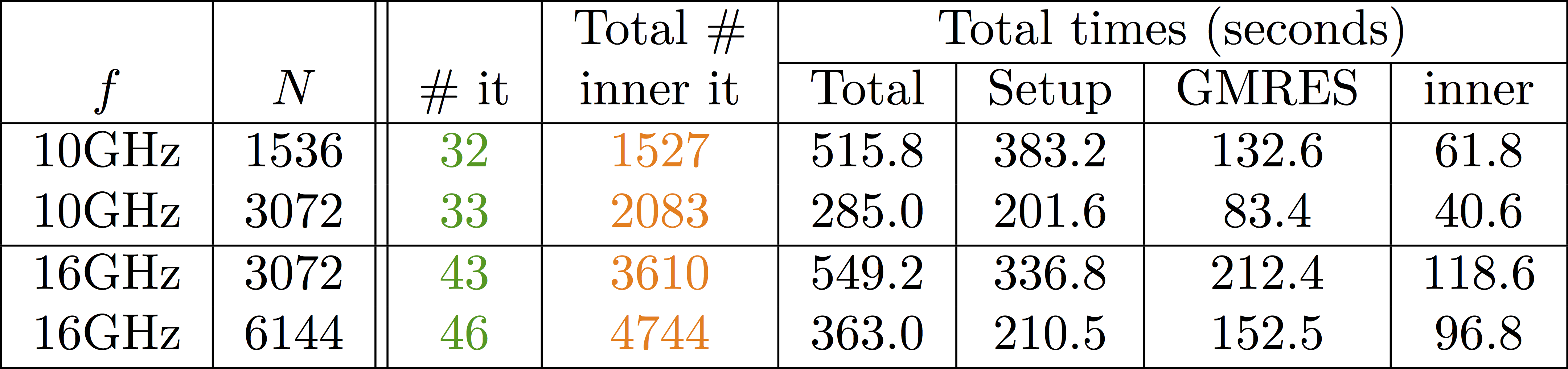speedup of 1.81 from 1536 to 3072 cores at 10GHz

1.51 from 3072 to 6144 cores at 16GHz

You can find the script here